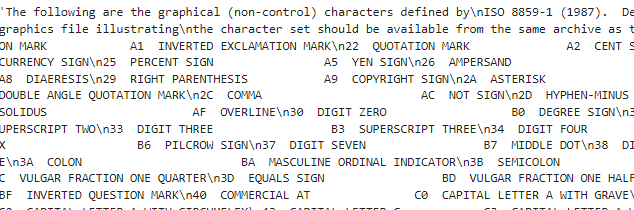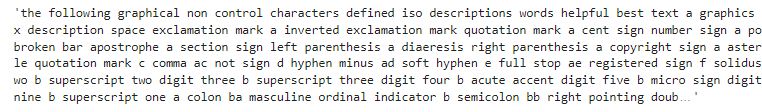Open in App
Not now

# Processing text using NLP | Basics

• Last Updated : 22 Sep, 2022

In this article, we will be learning the steps followed to process the text data before using it to train the actual Machine Learning Model.

## Importing Libraries

The following must be installed in the current working environment:

• NLTK Library: The NLTK library is a collection of libraries and programs written for processing of English language written in Python programming language.
• urllib library: This is a URL handling library for python.
• BeautifulSoup library: This is a library used for extracting data out of HTML and XML documents.

## Python3

 `import` `nltk``from` `bs4 ``import` `BeautifulSoup``from` `urllib.request ``import` `urlopen`

Once importing all the libraries, we need to extract the text. Text can be in string datatype or a file that we have to process.

## Extracting Data

For this article, we are using web scraping to read a webpage then we will be using get_text() function for changing it to str format.

## Python3

 `raw ``=` `urlopen(``"https://www.w3.org/TR/PNG/iso_8859-1.txt"``).read()` `raw1 ``=` `BeautifulSoup(raw)``raw2 ``=` `raw1.get_text()``raw2`

Output :## Data Preprocessing

Once the data extraction is done, the data is now ready to process. For that follow these steps :

## Python3

 `# deletion of punctuations and numerical values``def` `punc(raw2):``  ``raw2 ``=` `re.sub(``'[^a-zA-Z]'``, ``' '``, raw2)``  ``return` `raw2`

## Python3

 `# extracting tokens``def` `token(raw2):``  ``tokens ``=` `nltk.word_tokenize(raw2)``  ``return` `tokens`

## Python3

 `# lowercase the letters``# removing stopwords``def` `remove_(tokens):``  ``final ``=` `[word.lower()``         ``for` `word ``in` `tokens ``if` `word ``not` `in` `stopwords.words(``"english"``)]``  ``return` `final`

## Python3

 `# Lemmatizing``from` `textblob ``import` `TextBlob` `def` `lemma(final):``  ``# initialize an empty string``  ``str1 ``=` `' '``.join(final)``  ``s ``=` `TextBlob(str1)``  ``lemmatized_sentence ``=` `" "``.join([w.lemmatize() ``for` `w ``in` `s.words])``  ``return` `final`

## Python3

 `# Joining the final results``def` `join_(final):``  ``review ``=` `' '``.join(final)``  ``return` `ans`

To execute the above functions refer this code :

## Python3

 `# Calling all the functions``raw2 ``=` `punc(raw2)``tokens ``=` `token(raw2)``final ``=` `remove_(tokens)``final ``=` `lemma(final)``ans ``=` `join_(final)``ans`

Output :My Personal Notes arrow_drop_up